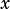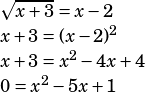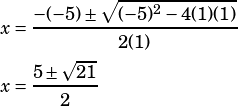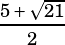Hi! For page 120 question 8 in the pwn book, is there any other way to find the answer without using a Calculator? I tried using a calculator but it took me a long time to find the answer. In addition, is there any other way to find that there is only one solution other than graphing or plugging the x values back into the equation?

To quickly answer your second question first, you should assume when you haveunder a square root sign that you’re going to have extraneous solutions. So in a question like this where the algebra gives you two solutions, you should assume only one of them really works.

To get this without using a calculator, use the quadratic formula.If you do it that way, you’ll actually see that only choice C could possibly work because the other choices have the wrong numbers in them. But if there were a choice that had both theandoptions, you’d still probably want to evaluate both with your calculator to see which is extraneous.